Ex 5.3

Chapter 5 Class 10 Arithmetic Progressions (Term 2)
Serial order wise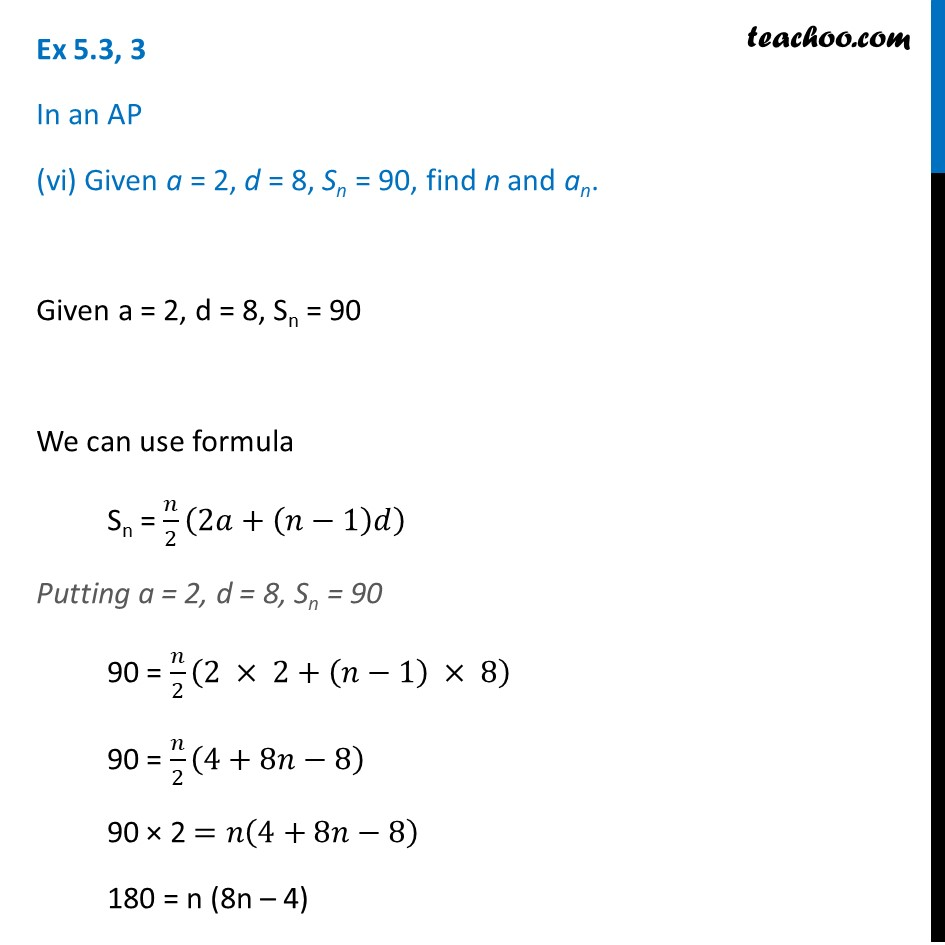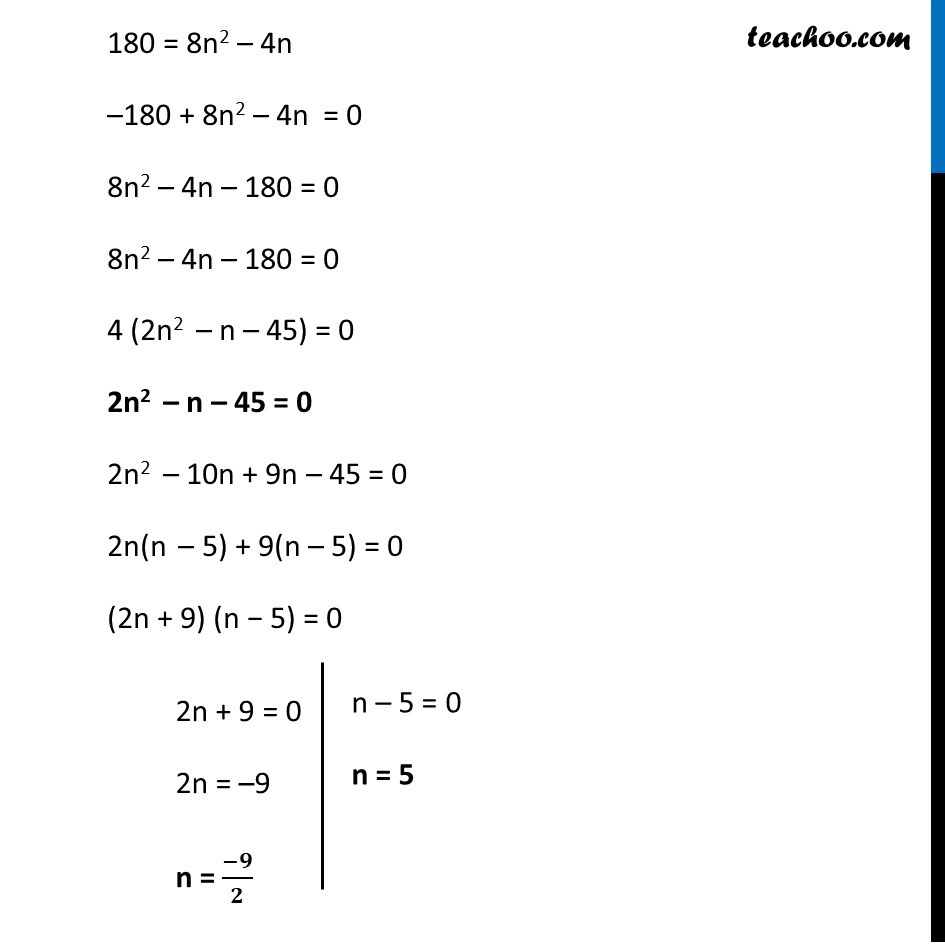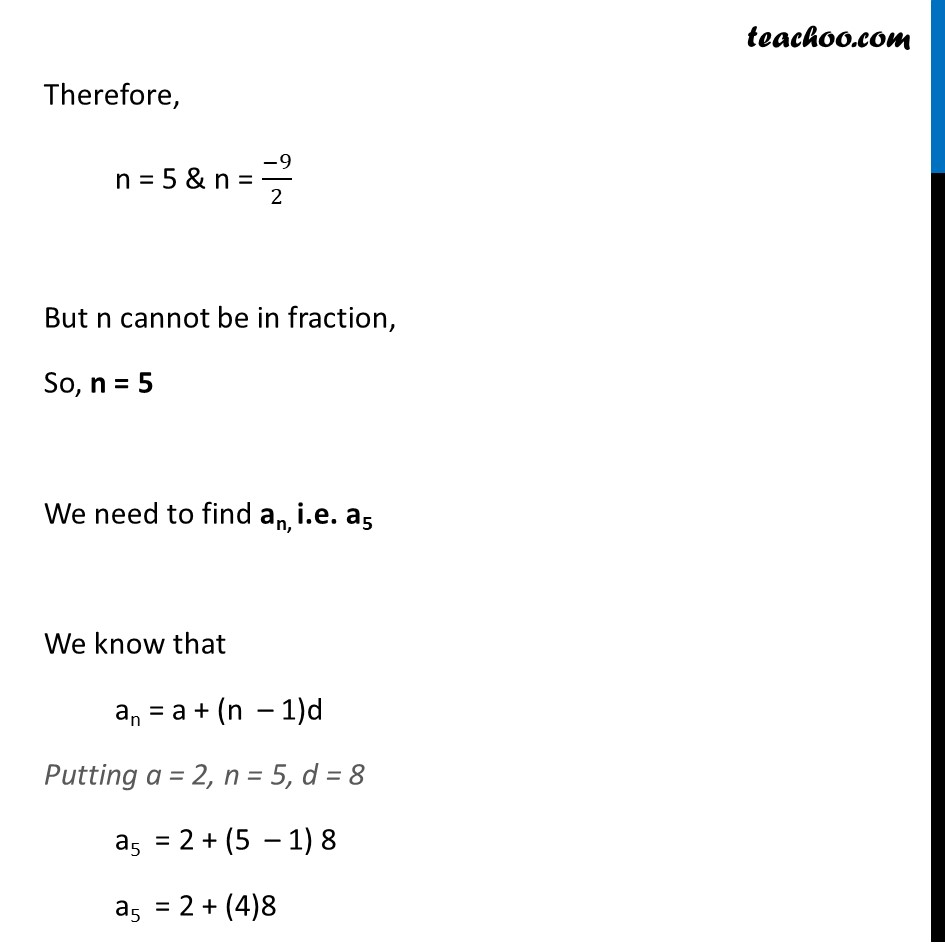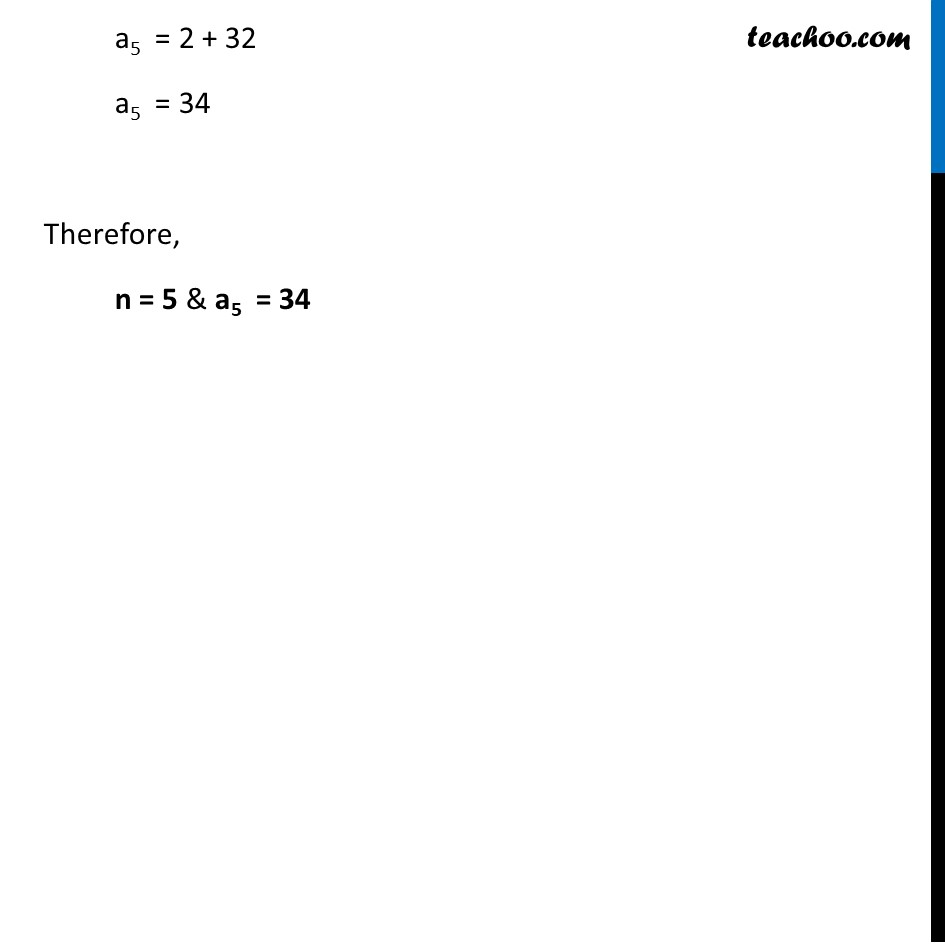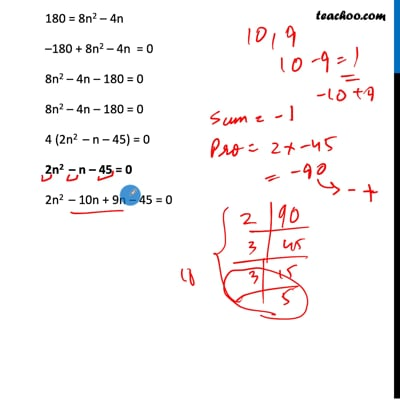This video is only available for Teachoo black users

### Transcript

Ex 5.3, 3 In an AP (vi) Given a = 2, d = 8, Sn = 90, find n and an. Given a = 2, d = 8, Sn = 90 We can use formula Sn = 𝑛/2 (2𝑎+(𝑛−1)𝑑) Putting a = 2, d = 8, Sn = 90 90 = 𝑛/2 (2 × 2+(𝑛−1) × 8) 90 = 𝑛/2 (4+8𝑛−8) 90 × 2 =𝑛(4+8𝑛−8) 180 = n (8n – 4) 180 = 8n2 – 4n –180 + 8n2 – 4n = 0 8n2 – 4n – 180 = 0 8n2 – 4n – 180 = 0 4 (2n2 – n – 45) = 0 2n2 – n – 45 = 0 2n2 – 10n + 9n – 45 = 0 2n(n – 5) + 9(n – 5) = 0 (2n + 9) (n − 5) = 0 2n + 9 = 0 2n = –9 n = (−𝟗)/𝟐 n – 5 = 0 n = 5 Therefore, n = 5 & n = (−9)/2 But n cannot be in fraction, So, n = 5 We need to find an, i.e. a5 We know that an = a + (n – 1)d Putting a = 2, n = 5, d = 8 a5 = 2 + (5 – 1) 8 a5 = 2 + (4)8 a5 = 2 + 32 a5 = 34 Therefore, n = 5 & a5 = 34